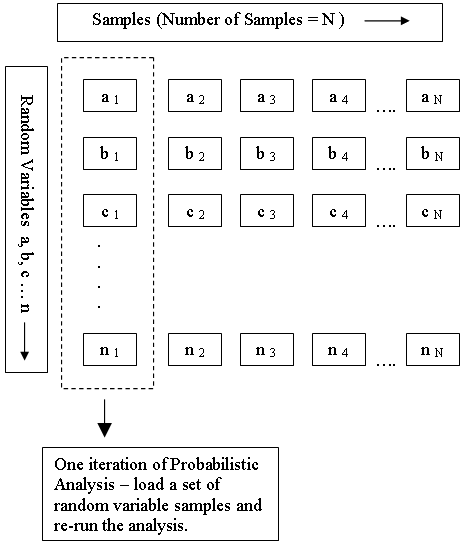# Random Variables

In order to carry out a Probabilistic analysis with RocPlane, you must define at least one input parameter as a random variable.

To define an input parameter as a random variable, select a Statistical Distribution for the variable (e.g., Normal, Uniform etc.) and enter the required statistical parameters (e.g. Mean Value, Standard Deviation, etc.). Input parameters that can be defined as random variables in a RocPlane Probabilistic analysis include:

In addition, if you are using the Joint Persistence Analysis option, you can define joint persistence as a random variable. For details, see Joint Persistence Analysis in a RocPlane Probabilistic Analysis.

For more information about the selection of Statistical Distributions and parameters, see Overview of Statistical Distributions in RocPlane.

## How are the Random Variables Used in the Analysis?

Each input parameter that is defined as a random variable in a Probabilistic analysis is sampled according to the Statistical Distribution selected for the variable as well as the Sampling Method and Number of Samples chosen in the Project Settings dialog (see Sampling Settings in RocPlane). This generates N values of each random variable (where N = Number of Samples).

As shown in the following diagram, each iteration of the Probabilistic analysis is carried out by loading a new set of random variable samples and re-running the analysis. This is repeated N times where N = Number of Samples.Random Variable Samples used in Probabilistic Analysis